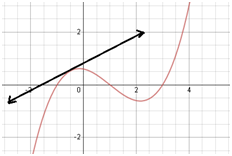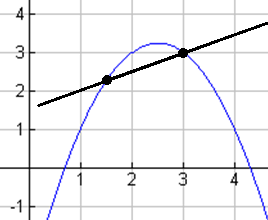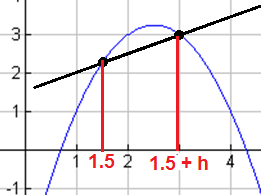# The Tangent Line Problem - Day 1 of 2

3 teachers like this lesson
Print Lesson

## Objective

SWBAT to find the slope of the tangent line to a function.

#### Big Idea

Limits and slope join forces to help solve a difficult problem.

1 minutes

The original lesson on this page is an introduction to tangent lines and derivatives without any context. When I taught this lesson this year, I wanted to start with a context right away so that students could see a need for derivatives and tangent lines. This alternate form of this lesson (the Rock Problem) is essentially the same as the original except that it is presented in a context - students are asked to find instantaneous velocity instead of instantaneous slope.

My students have a lot of experience with velocity from physics, so I knew that this context would be appropriate for them. If your students do not have that prior knowledge, then it might be okay to stick with the original task. In this document are some hints on how I can get students thinking about slope and limits to complete the Rock Problem task successfully.

All of the student work on this lesson page is from the Rock Problem instead of the original task.

## Launch

5 minutes

Today's lesson is extremely important - students will be introduced to the derivative of a function. The derivative is something that will become indispensable when students take calculus, so I want to make sure that they have a good conceptual understanding of it. I preface this lesson by telling students that what we learn over the next two days will be an important tool that they will be using all of the time in their math class next year.

I introduce derivatives in the context of the slope of a tangent line, so it is important that students know what a tangent line is. I give students this worksheet and we discuss #1 together. Most students remember a tangent line from their study of circles in Geometry, so I start by revisiting that definition.Teacher Note: Many resources will say that a tangent line intersects a curve at one point - that definition can be very confusing for students with a function like the one above. Yes, the tangent line only intersects one point on that portion of the graph, but it will eventually intersect other point on the function. Be sure to clear this up with students or they may not think that this is actually a tangent line. It might be helpful to describe the tangent line as the instantaneous slope of the curve.

## Explore

10 minutes

Once students understand what the definition of a tangent line is, I ask them to sketch the tangent line at x = 1.5 for the function shown for #2 on their worksheet. Then, I instruct them to find the slope of the tangent line. Like it says on the worksheet, if students cannot find the exact slope then I want them to find the best approximation they can. I will give students about 10 minutes or so to work on this problem with their table groups. Here are a few strategies that I usually notice my students use:

• Using another point on the tangent line: This is usually the most common strategy - students will estimate another point on the tangent line that they sketched in. Then they will find the slope of the tangent line using those two points.
• Using another point on the function: Some students will choose an x-value like 1.6, and find the point on the function. Then they will find the slope of the line that connects those two points. It is not the exact slope of the tangent line, but it is close.
• Using a perpendicular line: Many students remember that in a circle, the tangent line is perpendicular to the radius of the circle. Some will extend this logic and decide that this tangent line must be perpendicular to another line, so they will try to sketch another line and find that slope to help them find the slope of the tangent line.

I don't expect my students to be able to find this answer on their own. Some may and that is great - but the main purpose is just to get students thinking about the slope of the tangent line and different ways that we can find it. I always want them to recognize that it is a tough problem because we only know one point that is for sure on the tangent line.

## Share

20 minutes

I always start our discussion by asking students what was difficult about this problem. Usually they will note that we are trying to find the slope of a line but we only know one point on the line instead of the two that are needed.

Today is rare in that I usually do not start with student work because there is a very specific direction I want the conversation to go in. I usually start by putting a point at (3, 3) on the graph and connecting it to the point (1.5, 2.25) as shown below.I ask a randomly selected student how this slope compares to the slope of the tangent line. They will notice that slope is less than what it should be. Then I will choose a new point closer to (1.5, 2.25) and ask about that slope. Students will generally see that as the second point gets closer to (1.5, 2.25) our slope is a better estimate of the slope of the tangent line. In the video below I show a visual that I created in Geogebra that is really important for your students.

At this point we will go through the limit method of finding the slope of the tangent line. I will usually start with a diagram such as the one below, and ask students to set up the slope formula with the ordered pairs (1.5, 2.25) and (1.5 + h, f(1.5 + h)). Then we will say that we must take the limit as h goes to zero and do the necessary algebraic steps. As we go through our work I will ask students clarifying questions to make sure they understand the process.## Summarize

10 minutes

To me - the big takeaway from today's lesson is the need for limits to find the slope of the tangent line. I will ask students why the limit was needed and we will talk about it together. I also remind students of when we found the area under a curve with limits and look at the similarities between these problems.

Finally, I will assign #3 from the worksheet as homework.